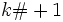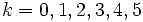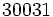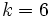# Euclid prime

This article defines a property that can be evaluated for a prime number. In other words, every prime number either satisfies this property or does not satisfy this property.
View other properties of prime numbers | View other properties of natural numbers

## Definition

A Euclid prime' is a prime number that is one more than a primorial. In other words, it is a prime number of the form, wheredenotes the product of the firstprimes.

A natural number of the formis termed a Euclid number, so a Euclid prime is a Euclid number that happens to be prime.

## Occurrence

The ID of the sequence in the Online Encyclopedia of Integer Sequences is A018239

### Initial values

The initial values of Euclid numbers are prime. For, the corresponding Euclid numbersare prime. The first Euclid number that is not prime is, corresponding to.